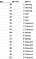# Simple project

## 0. Data and notebooksIt’s literally this simple 😅

## 1. Import the data

`import pandas as pd`
`import pandas as pddf = pd.read_csv(‘music.csv’)`

## 2. Prepare the data

`X = df.drop(columns=[‘genre’])y = df[‘genre’]`

## 3. Training and testing sets

`from sklearn.model_selection import train_test_splitX_train, X_test, y_train, y_test = train_test_split(X, y, test_size=0.2)`

## 5. Train & make predictions

`from sklearn.tree import DecisionTreeClassifiermodel = DecisionTreeClassifier()`
`model.fit(X_train, y_train)`

## 6. Test & tune

`predictions = model.predict(X_test)`
`from sklearn.metrics import accuracy_scoreaccuracy.score(y_test, predictions)`

# More complex project

## Data and libraries

`df.shapedf.isna().sum()df = df.dropna(axis=1)`

## Manipulating the data

`LabelEncoder_Y = LabelEncoder()df.iloc[:,1] = LabelEncoder_Y.fit_transform(df.iloc[:,1].values)`
`X = df.iloc[:,2:31].valuesy = df.iloc[:,1].values`

## More models

`#1 Logistic regressionfrom sklearn.linear_model import LogisticRegressionlog = LogisticRegression(random_state = 0)log.fit(X_train, y_train)#2 Decission treefrom sklearn.tree import DecisionTreeClassifiertree = DecisionTreeClassifier(criterion = ‘entropy’, random_state = 0)tree.fit(X_train, y_train)#3 Random forestfrom sklearn.ensemble import RandomForestClassifierforest = RandomForestClassifier(n_estimators = 10, criterion=’entropy’, random_state=0)forest.fit(X_train, y_train)`

# Bye & build

IT’S TIME TO BUILD!

Ambitious teenager building innovative projects with Synthetic Biology and Artificial Intelligence

## More from Sofia Sanchez

Ambitious teenager building innovative projects with Synthetic Biology and Artificial Intelligence